# CBSE Class 10 Science Previous Year Question Paper Term 1 2021 JSK-1-Set-4

The thing with CBSE class 10 science is that: either you are very good at it or you struggle with it a lot. In either way, we can help you score more in CBSE Class 10 Board exams. Have you taken a good look at the syllabus?

CBSE Class 10 Science highlights important concepts which include chemical reactions, principles, equations and a lot more. TopperLearning provides study materials for CBSE Class 10 Science which offers a systematic way to prepare for the final examination. Take our study notes, for example.

The CBSE Class 10 science notes summarize all the key points of a particular chapter in the syllabus so that you can glance through them any time you want. It’s the easiest way to revise the key learnings of every chapter in the class 10 syllabus for CBSE science without even opening your Class 10 science book.

Question 1.

A student while burning a magnesium ribbon in air, collected the products in a wet watch glass. The new product obtained was:

1. Magnesium oxide
2. Magnesium carbonate
3. Magnesium hydroxide
4. Magnesium chloride

Question 2.

Consider the following chemical equation:

2NaOH + H2SO4 ⟶ Na2SO4 + 2H2O

The information conveyed by this equation are:

NaOH reacts with H2SO4 to produce Na2SO4 and water.

For every one molecule of H2SO4, two molecules of NaOH are required.

Acids and bases are non-ionic in nature.

This is not a redox reaction.

The correct statements are:

1. I and II
2. II and III
3. III and IV
4. I and IV

Question 3.

Select the correct matching in the following table in connection with the given chemical reaction:

CuSO4 + Fe ⟶ FeSO4 + Cu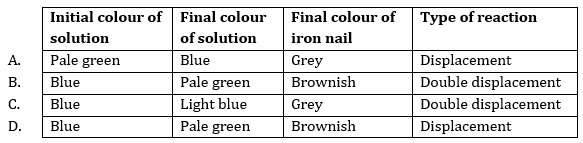Question 4.

Consider the following processes:

(i) Dilution of sulphuric acid

(ii) Sublimation of dry ice

(iii) Condensation of water vapours

(iv) Dissolution of ammonium chloride in water

The endothermic process(es) is/are :

1. I and III
2. II only
3. III only
4. II and IV

Question 5.

A solution gives yellowish orange colour when a few drops of universal indicator are added to it. This solution is of:

1. Lemon juice
2. Sodium chloride
3. Sodium hydroxide
4. Milk of magnesia

Question 6.

Concentrated H2SO4 is diluted by adding drop by drop:

1. Water to acid with constant stirring
2. Acid to water with constant stirring
3. Water to acid followed by a base
4. Base to acid followed by cold water

Question 7.

Absence of tartaric acid in baking powder makes the taste of the cake bitter due to the presence of:

1. Sodium hydrogen carbonate
2. Sodium carbonate
3. Sodium metabisulphite
4. Sodium sulphate

Question 8.

The pH of acid rain is approximately:

1. 5.6
2. 6.4
3. 7.0
4. 7.9

Question 9.

Salt 'A’ commonly used in food products, is a reactant to produce salt ‘B', used in the kitchen for making tasty, crispy pakoras. Salt ‘B’ on heating converts into another salt 'C’, which is used in the manufacturing of glass. Salts ‘A', ‘B' and 'C’ respectively are:
1. NaHCO3, NaCI, Na2CO3
2. Na2CO3, NaHCO3, NaCl
3. Na2CO3, NaCl, NaHC3
4. NaCl, NaHCO3, Na2,CO3

Question 10.
In the following diagram, what would happen if some amount of sodium hydroxide is taken in place of sulphuric acid and the test tube is heated: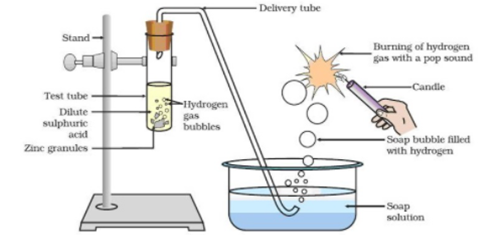1. Same amount of H2 gas is evolved
2. Ha gas is not evolved
3. The amount of H2 gas evolved is much less
4. In place of H2 gas, O2 gas evolves

Question 11.

In Human Alimentary canal, the specific enzyme/juice secreted in locations (i), (ii) and (iii) are: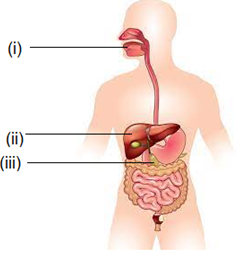1. (i) Amylase (ii) Pepsin (iii) Bile
2. (i) Amylase (ii) Bile (iii) Trypsin
3. (i) Lipase (ii) Amylase (iii) Pepsin
4. (i) Trypsin (ii) Bile (iii) Amylase

Question 12.

The sequence of anaerobic respiration in our muscle cells during heavy exercise is: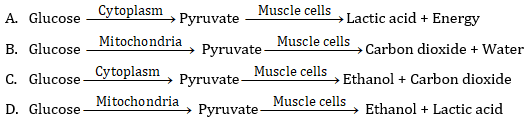Question 13.

The separation of the right side and the left side of human heart is useful to:
1. Keep oxygenated blood from mixing with deoxygenated blood
2. Allow a slow supply of oxygen in the body
3. Supply energy to animals with low energy needs
4. Often change their body temperature

Question 14.

In spring, sugar stored in root or stem tissue of plants is transported to the buds for:
1. The energy needs of the buds to grow
2. Temperature regulation
3. Balancing the storage in different organs
4. Diffusion process

Question 15.

The energy released during cellular respiration is used to synthesize:
1. Ribosomes
2. RBC
3. ATP
4. Mitochondria

Question 16.

Upward movement of water in tall trees is due to:
1. Translocation
2. Excretion
3. Photosynthesis
4. Transpiration

Question 17.

If a lens and a spherical mirror both have a focal length of -15 cm, then it may be concluded that:
1. Both are concave
2. The lens is concave and the mirror is convex
3. The lens is convex and the mirror is concave
4. Both are convex

Question 18.

An optical device forms an erect image of an object placed in front of it. If the size of the image is one half that of the object, the optical device is a:

1. Concave mirror
2. Convex mirror
3. Plane mirror
4. Convex lens

Question 19.

A student determines the focal length of a device 'A' by focussing the image of a far off object on a screen placed on the opposite side of the object. The device 'A' is:

1. Concave lens
2. Concave mirror
3. Convex lens
4. Convex mirror

Question 20.

When light is incident on a glass slab, the incident ray, refracted ray and the emergent ray are in three media A, B and C. If n1, n2 and n3 are the refractive indices of A, B and C respectively and the emergent ray is parallel to the incident ray, which of the following is true?

1. n1 < n2 < n3
2. n1 > n2 > n3
3. n1 < n2 = n3
4. n1 = n3 < n2

Question 21.

The image of a candle flame formed by a lens is obtained on a screen placed on the other side of the lens. According to new Cartesian sign convention, if the image is three times the size of the flame, then the lens is:

1. Concave and magnification is + 3
2. Concave and magnification is - 3
3. Convex and magnification is - 3
4. Convex and magnification is + 3

Question 22.

The power of a combination of two lenses in contact is + 1.0 D. If the focal length of one of the lenses of the combination is + 20.0 cm, the focal length of the other lens would be :

1. -120-0 cm
2. +80-0 cm
3. – 25.0 cm
4. – 20.0 cm

Question 23.

When a ray of light passes through a glass prism it suffers two refractions. During these refractions the ray bends:

1. Away from the base in both cases.
2. Towards the base cases in both cases.
3. Towards the base in first case and away from the base in second case.
4. Away from the base in first case and towards the base in second case.

Question 24.

To an astronaut the sky on the moon appears dark because:

1. There is no light on the moon.
2. There is no atmosphere on the surface of the moon.
3. Moon is a non-luminous object.
4. The surface of the moon absorbs all the sunlight.

SECTION – B

Section - B consists of 24 questions (Sl. No.25 to 48). Attempt any 20 questions from this section.

The first attempted 20 questions would be evaluated.

Question 25.

When lead nitrate powder is heated in a boiling tube, we observe:

1. Brown fumes of nitrogen dioxide
2. Brown fumes of lead oxide
3. Yellow fumes of nitrogen dioxide
4. Brown fumes of nitric oxide

Question 26.

An aqueous solution of a salt shows an orange red colour when a drop of universal indicator is added to it. This salt is made up of:

1. A strong acid and a strong base
2. A weak acid and a weak base
3. A strong acid and a weak base
4. A weak acid and a strong base

Question 27.

Three test tubes A, B and C contain distilled water, an acidic solution and a basic solution respectively. When red litmus solution is used for testing these solutions, the observed colour changes respectively will be:

1. A — no change; B — becomes dark red; C — becomes blue
2. A — becomes light red; B — becomes blue; C — becomes red
3. A — becomes red; B — no change; C — becomes blue
4. A — becomes light red; B — becomes dark red; C — becomes blue

Question 28.

Given below is a reaction showing Chlor-alkali process:

2NaC1 (aq) + 2H2O (l) ⟶ 2NaOH (aq) + Cl2 (g) + H2 (g)

(A)             (B)         (C)

The products A, B and C are produced respectively:

1. At the anode, at the cathode, near the cathode.
2. Near the cathode, at the anode, at the cathode.
3. At the cathode, near the cathode, at the anode.
4. At the anode, near the cathode, at the cathode.

Question 29.

In the activity shown in the diagram, if the climate is humid, the role of calcium chloride taken in the guard tube is to: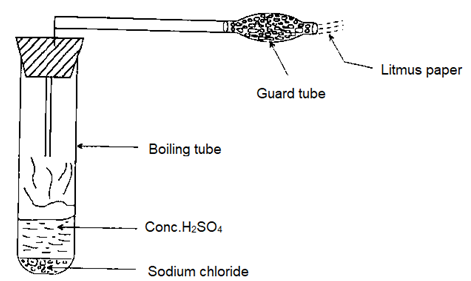1. Absorb the evolved gas
2. Warm up the gas
3. Dry the gas .V
4. Absorb chloride ions from the evolved gas.

Question 30.

Which one of the following chemicals is used in soda acid fire extinguishers?

1. Sodium chloride
2. Sodium acetate
3. Sodium hydrogen carbonate
4. Ammonium sulphate

Questions No. 31 to 34 consists of two statements — Assertion (A) and Reason (I?). Answer these questions selecting the appropriate options given below:

1. Both Assertion (A) and Reason (R) are true and Reason (R) is the correct explanation of Assertion (A).
2. Both Assertion (A) and Reason (R) are true, but Reason (R) is not the correct explanation of Assertion (A).
3. Assertion (A) is true, but Reason (R) is false.
4. Assertion (A) is false, but Reason (R) is true.

Question 31.

Assertion (A) : Silver salts are used in black and white photography.

Reason (R) : Silver salts do not decompose in the presence of light.

Question 32.

Assertion (A) : The solutions of ionic compounds are good conductors of electricity.

Reason (R) : Movement of atoms of elements take place in solution.

Question 33.

Assertion (A) : The rate of breathing in aquatic organisms is much slower than that seen in terrestrial organisms.

Reason (R) : The amount of oxygen dissolved in water is very low as compared to the amount of oxygen in air.

Question 34.

Assertion (A) : Kerosene having higher refractive index is optically denser than water, although its mass density is less than that of water.

Reason (R) : The speed of light decides whether a medium is optically rarer or optically denser. An optically denser medium may not possess greater mass density.

Question 35.

Match the metal (Column I) with its reaction with oxygen (Column II) :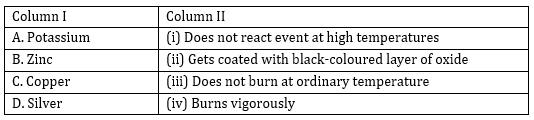1. A-(iv), B-(iii), C- (ii), D-(i)
2. A-(iv), B-(ii), C-(i), D-(iii)
3. A-(iii), B-(ii), C-(i), D-(iv)
4. A-(iv), B-lii), C-(iii), D-(i)

Question 36.

Four test tubes A, B, C and D are taken. In test tube A iron nail is dipped in copper sulphate solution. In test tube B copper wire is dipped in ferrous sulphate solution. In test tube C zinc metal is dipped in ferrous sulphate solution and in test tube D iron nail is dipped in zinc sulphate solution. The reactivity order has been found to be Zn > Fe > Cu. In which test tubes was the colour change observed?

1. A and C
2. A and B
3. B and C
4. B and D

Question 37.

The function not performed by villi is:

1. To increase the surface area for absorption
2. To ensure rich supply of blood vessels
3. Absorption of food
4. Egestion of food

Question 38.

In the given diagram, A, B, C and D respectively are: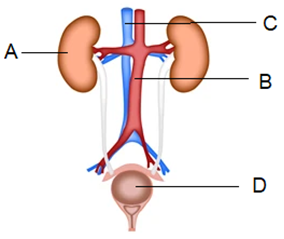1. A - Left kidney; B - Aorta; C - Vena cava; D - Urethra
2. A - Left kidney; B - Vena cava; C - Aorta; D - Urinary bladder
3. A - Right kidney; B - Aorta; C - Ureter; D - Urethra
4. A - Right kidney; - Vena cava; C - Aorta; D - Urinary bladder

Question 39.

Thin walled blood vessels are called:

1. Aorta
2. Capillaries
3. Arteries
4. Vena cava

Question 40.

The function of the lining of mucus in the nasal passage of human beings is to :

1. Increase the temperature of inhaled air
2. Move the air in and out
3. Filter the air that we breathe in
4. Absorb oxygen from the air

Question 41.

In the following flow chart showing autotrophic nutrition in green plants, A and B respectively are: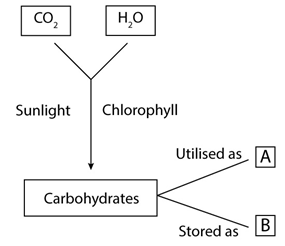1. Oxygen and energy
2. Starch and oxygen
3. Energy and starch
4. Oxygen and water

Question 42.

Study the diagram given below and identify the type of the lens XX' and the position of the point on the principal axis OO' where the image of the object AB appears to be formed :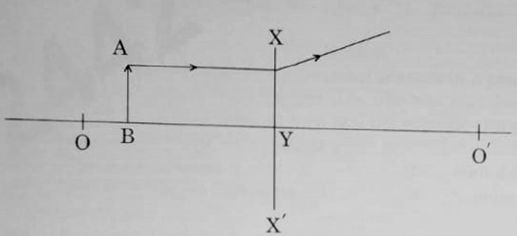1. Concave; between O' and Y
2. Concave; between 0 and Y
3. Convex; between O' and Y
4. Convex; between 0 and Y

Question 43.

The image of an object placed in front of a concave mirror of focal length 15 cm is of the same size as the object. The distance between the object and its image is:

1. 15 cm
2. 30 cm
3. 60 cm
4. Zero

Question 44.

An object of height 3.0 cm is placed vertically on the principal axis of a convex lens. When the object distance is - 37.5 cm, an image of height - 2.0 cm is formed at a distance of 25.0 cm from the lens. Next, the same object is placed vertically at 25.0 cm from the lens. In this situation the image distance v and height h of the image is (according to the new Cartesian sign convention):

1. v = + 37-5 cm; h = + 4.5 cm
2. v = - 37.5 cm; h = + 4.5 cm
3. v = + 37-5 cm; h = - 9-5 cm
4. v = - 37.5 cm; h = - 4.5 cm

Question 45.

The relation R = 2f is valid:

1. For concave mirrors but not for convex mirrors
2. For convex mirrors but not for concave mirrors
3. Neither for concave mirrors nor for convex mirrors
4. For both concave and convex mirrors

Question 46.

A lens has a power of + 4.0 D. It is:

1. A convex lens of focal length 4 m
2. A concave lens of focal length 4 m
3. A convex lens of focal length 0.25 m
4. A concave lens of focal length 0.25 m

Question 47.

An object is placed in front of a concave lens. For all positions of the object the image formed is always:

1. Real, diminished and inverted
2. Virtual, diminished and erect
3. Real, enlarged and erect

Question 48.

Virtual, erect and enlarged. The twinkling of star is due to:

1. Scattering of light
2. Dispersion of light
3. Tyndall effect
4. Atmospheric refraction

SECTION – C

Section- C consists of three Cases followed by questions. There are a total of 12 questions in this section. Attempt any 10 questions from this section.

The first attempted 10 questions would be evaluated.

Case I: One day Kamal saw that her mother was roasting peanuts in a pan (kadahi) in the kitchen and she had taken ordinary salt to roast the peanuts. She was surprised to observe that in spite of very high temperature, the salt does not melt and the peanuts also get roasted.

Question 49.

The chemical name of common salt is:

1. Potassium chloride
2. Sodium carbonate
3. Sodium hydrogen carbonate
4. Sodium chloride

Question 50.

Common salt is:

1. A covalent compound
2. An ionic compound formed by sharing electrons
3. An ionic compound formed by the transfer of electrons
4. Soluble in organic solvents

Question 51.

Common salt is not a raw material for:

1. Bleaching powder
2. Plaster of Paris
3. Baking soda
4. Caustic soda

Question 52.

Common salt is used as a raw material in the preparation of washing soda in which the number of molecules of water of crystallisation is:

1. 10

Case II:

Activity: Take a healthy potted plant with elongated leaves. Select a leaf and insert about one half of this leaf in a test tube containing KOH and make it air tight. Place the set-up in sun for two hours. Take out the leaf-from the test tube and dip it in boiling water for a few minutes. Put this leaf in a beaker with alcohol and boil it in a water bath. Wash the leaf with water and then dip the leaf in iodine solution for a few minutes. The portion of the leaf dipped in KOH solution will not show any change when dipped in iodine solution.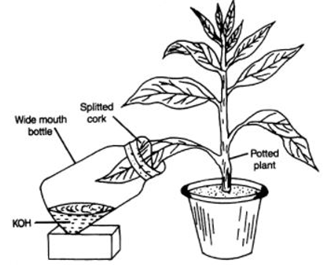Question 53.

The function of KOH taken in the test tube is to absorb:

1. Released water vapours
2. Released CO2
3. Released O2
4. Chlorophyll

Question 54.

On the basis of this activity, we may conclude that the essential factor for photosynthesis is:

1. Carbon dioxide
2. Oxygen
3. Chlorophyll
4. Water vapour

Question 55.

The event that does not occur in photosynthesis is:

1. Absorption of light energy by chlorophyll.
2. Reduction of carbon dioxide to carbohydrates.
3. Oxidation of carbon to carbon dioxide.
4. Conversion of light energy to chemical energy.

Question 56.

Iodine solution gives blue-black colour with:

1. Starch
2. Proteins
3. Glucose
4. Fats

Case III: On a sunny day, Krish looked at the sky through a water fountain and was surprised to see a rainbow in the sky.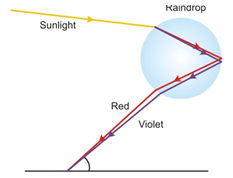Question 57.

The location of the sun when Krish observed a rainbow was:

1. Behind him
2. In front of him
4. On his left side

Question 58.

The phenomena of light involved in the formation of a rainbow are:

1. Reflection, refraction, dispersion
2. Refraction, dispersion, internal reflection
3. Refraction, dispersion, scattering
4. Dispersion, scattering, internal reflection

Question 59.

In the formation of a rainbow, the role of water droplets presents in the water fountain is to act as a:

1. Glass slab
2. Convex lens
3. Concave lens
4. Prism

Question 60.

While entering a water droplet the sunlight gets:

1. Refracted only
2. Reflected internally
3. Refracted and dispersed
4. First refracted and then dispersed while coming out of the water droplet.

Ans 1.  Correct option – c : Magnesium hydroxide When a magnesium ribbon is burned in air, it burns with a dazzling white flame producing white ash of MgO. If the produced ash is collected in a wet watch glass then MgO will dissolved in water producing Magnesium hydroxide. Reaction:  MgO +H2O ⟶ Mg(OH)2

Ans 2.   Correct option – d : I and IV Statement I and IV are correct statements. 2aOH + H2SO4 ⟶ Na2SO4 + 2H2O In the above reaction NaOH reacts with H2SO4 to produce Na2SO4 and water. And this is not a redox reaction. It is an example of neutralization reaction.

Ans 3.  Correct option – d : Blue: Pale green : Brownish: Displacement CuSO4 + Fe ⟶ FeSO4 + Cu When an iron nail is dipped in copper sulphate solution, a brown coating of copper is formed in the surface of iron and the colour of copper sulphate solution changes from blue to pale green. The reaction shows iron displaces copper from the copper sulphate solution hence it is an example of displacement reaction.

Ans 4.  Correct option – b : II only Sublimation of dry ice required energy so it is endothermic process.

Ans 5.  Correct option – a : Lemon juice An acidic solution gives yellowish orange colour when a few drops of universal indicator added to it. Among the given options lemon juice is acidic.

Ans 6.  Correct option – b : Acid to water with constant stirring Concentrated acid is diluted by adding drop by drop acid to water with constant stirring.

Ans 7.  Correct option – b : sodium carbonate If tartaric acid is not added in baking powder, the sodium carbonate remains in the cake resulting in a bitter taste.

Ans 8.  Correct option – a : 5.6 The pH of the acid rain drops below 5.6.

Ans 9.  Correct option – a : NaHCO3, NaCl, Na2CO3 ′A′ is Sodium hydrogen carbonate (baking soda), ‘B’ is NaCl and salt ‘C’ is Na2CO3

Ans 10.  Correct option – a : same amount of H2 gas is evolved. If sodium hydroxide is taken in place of dilute sulphuric acid and the tube is heated then the metal will react with the base to form the corresponding salt and will evolve hydrogen gas as per the following reaction:-  Zn + 2NaOH → Na2ZnO2 + H2 .

Ans 11.  Correct option – b : (i) Amylase (ii) Bile (iii) Trypsin Salivary glands secrete salivary amylase, liver secretes bile while pancreas secretes pancreatic juice containing trypsin.

Ans 12.  Correct option – a :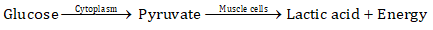Anaerobic respiration in human muscle cells during heavy exercise involves the breakdown of glucose in the cytoplasm to release pyruvate which is further broken down in the muscle cells to produce lactic acid and ATP energy.

Ans 13.

Correct option – a : Keep oxygenated blood from mixing with deoxygenated blood.

The right and left sides of the heart are separated by a muscular wall that prevents blood without oxygen from mixing with blood that has oxygen.

Ans 14.

Correct option – a : The energy needs of the buds to grow

During spring season, when the plants produce buds, more energy is needed in the bud region so as to bloom fully.

Ans 15.

Correct option – c : ATP

During cellular respiration, the energy released, is immediately used to synthesize a molecule known as ATP. ATP is further used to fuel all other activities in the cell.

Ans 16.

Correct option – d : Transpiration

Transpiration is the driving force behind uptake and transport of water in tall trees.

Ans 17.

Correct option – a: Both are concave

According to the New cartesian sign convention, concave mirror and concave lens are denoted by negative focal length. Thus, both the lens and spherical mirror are concave.

Ans 18.

Correct option – b: convex mirror

It is given that image formed is half of that of object. Thus, image is diminished. The erect and diminished image is possible only by using convex mirror.

Ans 19.

Correct option – c: convex lens

It is given that the student determines focal length by focusing the image on the screen. As the image can be obtained on screen it means that the image formed is real and inverted. It is also given that the image is formed in the opposite side of object. So, such type of image can be obtained only by using convex lens.  So, device ‘A’ is convex lens.

Ans 20.

Correct option – d: n1 = n3 < n2

When the ray of light is incident on glass slab then all rays i.e., incident, refracted and emergent are in three media. It is given that the emergent rays are parallel to incident this indicates that 1st and 3rd media are same. Thus, refractive index of both these media will be equal. And glass slab is denser media thus the refractive index of this media i.e., n2 will be greater than n1 and n3.

Ans 21.

Correct option – c: Convex and magnification is – 3

It is given that the image is formed on the screen. This means that image obtained is real and inverted. It is also given that the image is three times the size of the object. Thus, the image formed is magnified. Thus, the image formed is real, inverted and magnified and to the other side of the lens. Thus, the lens used is convex lens and as the image is inverted and magnified the magnification will be – 3.

Ans 22.

Correct option – c: – 25.0 cm

Power of combination of two lenses in contact is +1 D.

Focal length of one lens is +20 cm. The positive sign indicates that one of the lenses is convex lens which has focal length 20 cm or 0.20 m.

We know,

Power of combination of lens, P = P1 + P2

Thus,

Focal length of combination of lenses,Ans 23.

Correct option – b: towards the base in both cases

When ray of light enters the prism, the ray of light is travelling from rarer medium to denser medium, thus, the ray of light will bend towards the normal and hence, towards the base of prism. When this ray of light is coming out of the prism i.e., from denser medium to rarer medium it will bend away from the normal i.e., again towards the base of the prism. Observe the diagram given below for the answer.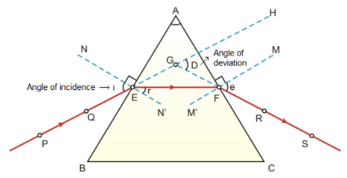Ans 24.

Correct option – b: There is no atmosphere on the surface of the moon

As there is absence of sunlight on the surface of the moon there is no scattering of light. Thus, sky on the moon appears dark to astronaut.

SECTION – B

Ans 25.

Correct option – a :   Brown fumes of nitrogen dioxide

It is a decomposaition reaction.

Lead nitrate decomposes on heating and gives a yellow residue of lead monoxide. Brown gas nitrogen dioxide and colourless gas oxygen are liberated.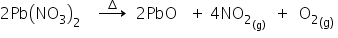Ans 26.

Correct option – c : Strong acid and weak base

Because solution should be weakly acidic than only universal indicator show orange colour.

Ans 27.

Correct option – a : A- no change; B- becomes dark red, C- becomes blue

Red litmus paper changes to blue in alkaline solution.

Ans 28.

Correct option – b : Near cathode, at the anode, at the cathode

During the electrolysis of sodium chloride solution chlorine gas is evolved at anode and hydrogen gas at cathode.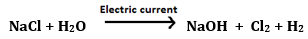Ans 29.

Correct option – c : Dry the gas

Due to the presence of humidity, hydrogen chloride gas may be lost as hydrochloric acid. To get anhydrous hydrogen chloride, CaCl2 is taken in a guard tube because it is a dehydrating agent and absorbs moisture.

Ans 30.

Correct option – c : Sodium hydrogen carbonate

Chemicals used in the Acid Fire Extinguisher are sodium hydrogen carbonate and dilute sulphuric acid. The gas evolved in the reaction is carbon dioxide.

Ans 31.

Correct option – c : A is true, but reason is wrong

Silver salts (AgBr and AgCl) are used in black and white photography. Silver salts undergoes decomposition in presence of sunlight.

2AgBr → 2Ag + Br

Ans 32.

Correct option – c : A is true, but reason is wrong

Solution of Ionic compounds are good conductors of electricity because of flow of ions. In any solution, movement of ions takes place so reason is wrong.

Ans 33.

Correct option – d : A is false, but R is true.

The rate of breathing in aquatic organisms is much faster than in terrestrial organisms because the amount of dissolved oxygen is fairly lower in water than air and also that aquatic organisms like the fishes obtain oxygen from water present in the dissolved state.

Ans 34.

Correct option – (A): Both Assertion (A) and Reason (R) are true and Reason (R) is the correct explanation of Assertion (A).

In the given assertion (A) a term optical density is used to describe the change in speed of light when it travels through a medium it can be compared to refractive index and as we know refractive index of kerosene is greater than water (i.e., 1.44 > 1.33) hence optical density of kerosene will be greater than water.

Also, we must note that mass density of material is not related with optical density.

Hence for the given case reason perfectly explain the assertion.

Ans 35.

Correct option –a : A-(iv), B-(iii), C-(ii), D-(i)

Ans 36.

Correct option – a:  A and C

If an iron nail is dipped in the copper sulphate solution then iron displaces copper from the copper sulphate because iron is more reactive than copper. Therefore the colour of the copper sulphate solution changes.

When zinc is added to the solution of iron sulphate, the colour of iron sulphate solution changes. It is because zinc is more reactive than iron, therefore, it displaces iron from its solution of iron sulphate and a grey precipitate of iron and a colourless solution of zinc sulphate is formed.

Ans 37.

Correct option – d : Egestion of food

The main function of villi is to increase the surface area of the small intestine wall which helps in absorption of the digested food. Blood vessels are also present within these villi, which helps to absorb the digested food and carry it to the bloodstream.

Ans 38.

Correct option – d : A – Right kidney; B – Venacava; C – Aorta; D – Urinary bladder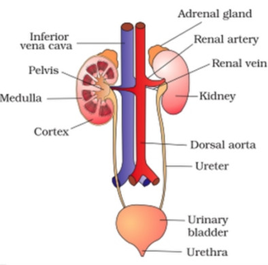Ans 39.

Correct option – b : Capillaries

Capillaries are tiny, extremely thin-walled vessels that act as a bridge between arteries (which carry blood away from the heart) and veins (which carry blood back to the heart).

Ans 40.

Correct option – c: Filter the air that we breathe in

The mucosal lining of the nasal passage helps prevent allergens and infections from invading the nasal cavity and spreading to other body structures.

Ans 41.

Correct option – c: Energy and starch

Carbohydrates in the form of glucose formed during photosynthesis are utilised to obtain energy in the form of ATP. Excess of glucose is stored in the form of starch in plants.

Ans 42.

Correct option – b: Concave; between O and Y

In the diagram, it is given that the ray after refraction is getting diverged. This indicates that it is concave lens. Thus, the image will be formed at the same side of the object i.e., between O and Y.

Ans 43.

Correct option - d: Zero

Given that,

Focal length, f = -15 cm

Size of object, h = size of image, h’

Now for the given concave mirror the magnification power can be given asHere, v and u are image distance and object distance for the given concave lens and h’ is considered as negative since image formed in inverted and long negative y-axis.

Alternate method:

The ray diagram for the given case can be illustrated as shown below and from this we conclude that if size of image and object are equal then the object and image distance will be same (i.e., R = 2f).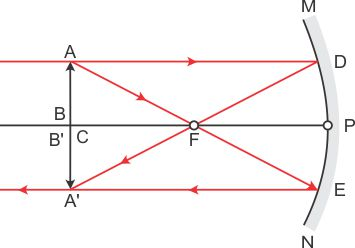Ans 44.

Correct option – c: v = +37.5 cm; h = - 4.5 cm

Using lens formula,On calculating, we get f = +15 cm

Now, the same object is at 25 cm in front of lens

Now, the focal length is 15 cm thus, twice the distance of the focal length will be 30 cm.

And as the object is placed at 25 cm it is in between the F and 2F on left side thus the image will be formed beyond 2F’ but to the right side of the lens. Thus, v = +37.5 cm.

Also in such cases, by convex lens the image formed will be inverted and magnified. Thus, h = - 4.5 cm

Ans 45.

Correct option – d: For both concave and convex mirror.

R= 2f is valid only for spherical (concave and convex) mirrors.

Ans 46.

Correct option – c: A convex lens of focal length 0.25 m

Focal length, f = 1/P = ¼ =0.25 m

Convex lens has positive focal length

Ans 47.

Correct option – b: Virtual, diminished and erect

Images formed by concave lens is always virtual, erect and diminished

Ans 48.

Correct option - d: Atmospheric refraction

The atmosphere is made up of several parallel layers of air with variable densities and refractive indices. The fluctuation in density between strata is caused by differences in temperature and pressure. As we ascend in height, the density and refractive index of the various strata decrease.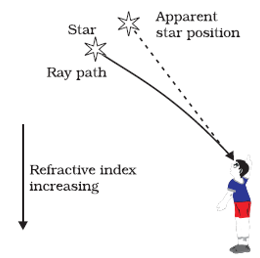Light from the stars is refracted numerous times as it travels through the many layers of the Earth's atmosphere. As a result, the star's light is bent (refracted) many times in random directions (light is bent when it hits a change in density - like a pocket of cold air or hot air).  The star twinkles as a result of this random refraction (it looks as though the star moves a bit, and our eye interprets this as twinkling).

Hence, we can conclude that, as a result of atmospheric refraction, the star twinkles.

SECTION – C

Ans 49.

Correct option – d : Sodium chloride

Chemical name of common salt (NaCl) is – Sodium chloride.

Ans 50.

Correct option – c : An ionic compound formed by transfer of electrons

Chemical formula of common salt is NaCl. So, we can say that it is made up of Na+ (metallic cation) and Cl (Non-metallic anion) and forms an ionic compound. Ionic compounds are formed due to transfer of electrons between cation and anion.

Ans 51.

Correct option – b : Plaster of Paris

Plaster of Paris is CaSO4.1/2 H2O. In its formula there is neither Na nor Cl so, you can say that it is not made up of Common salt (NaCl).

Ans 52.

Correct option – a :10

Formula of washing soda- Na2CO3.10H2O. So, water of crystallization is 10.

Ans 53.

Correct option – b : Released CO2

Potassium hydroxide (KOH) absorbs carbon dioxide which is necessary for photosynthesis.

Ans 54.

Correct option – a : Carbon dioxide

The given experiment is also known as Moll's half- leaf experiment which is being conducted to show that carbon dioxide is very much essential for the conduction of photosynthesis.

Ans 55.

Correct option – c : Oxidation of carbon to carbon dioxide

Photosynthesis does not involve the process of oxidation of carbon to carbon dioxide.

Ans 56.

Correct option – a : Starch

When there is presence of starch in the sample solution, the colour of the solution turns bluish black after the addition of the iodine solution.

Ans 57.

Correct option - a: Behind him

Rainbows are only visible in the sky when the sun is behind us, since light reflects inside water droplets as we can see from the given figure.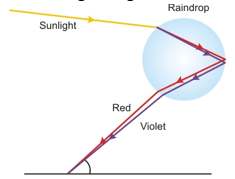Ans 58.

Correct option - b: Refraction, dispersion and internal reflection

Rainbows are formed by three phenomena: refraction, dispersion, and total internal reflection.

In the given example, the light bends slightly as it enters the water droplet due to a change in medium. This phenomenon is also known as refraction. Due to the slowing of light, white light is now split into seven various colours, and this light ray experiences total internal reflection when it reaches the other end of the droplet.

Ans 59.

Correct option - d: Prism

As we know just like prism water droplet can disperse white light into seven colour.

Hence option d is only correct among all.

Ans 60.

Correct option - c: